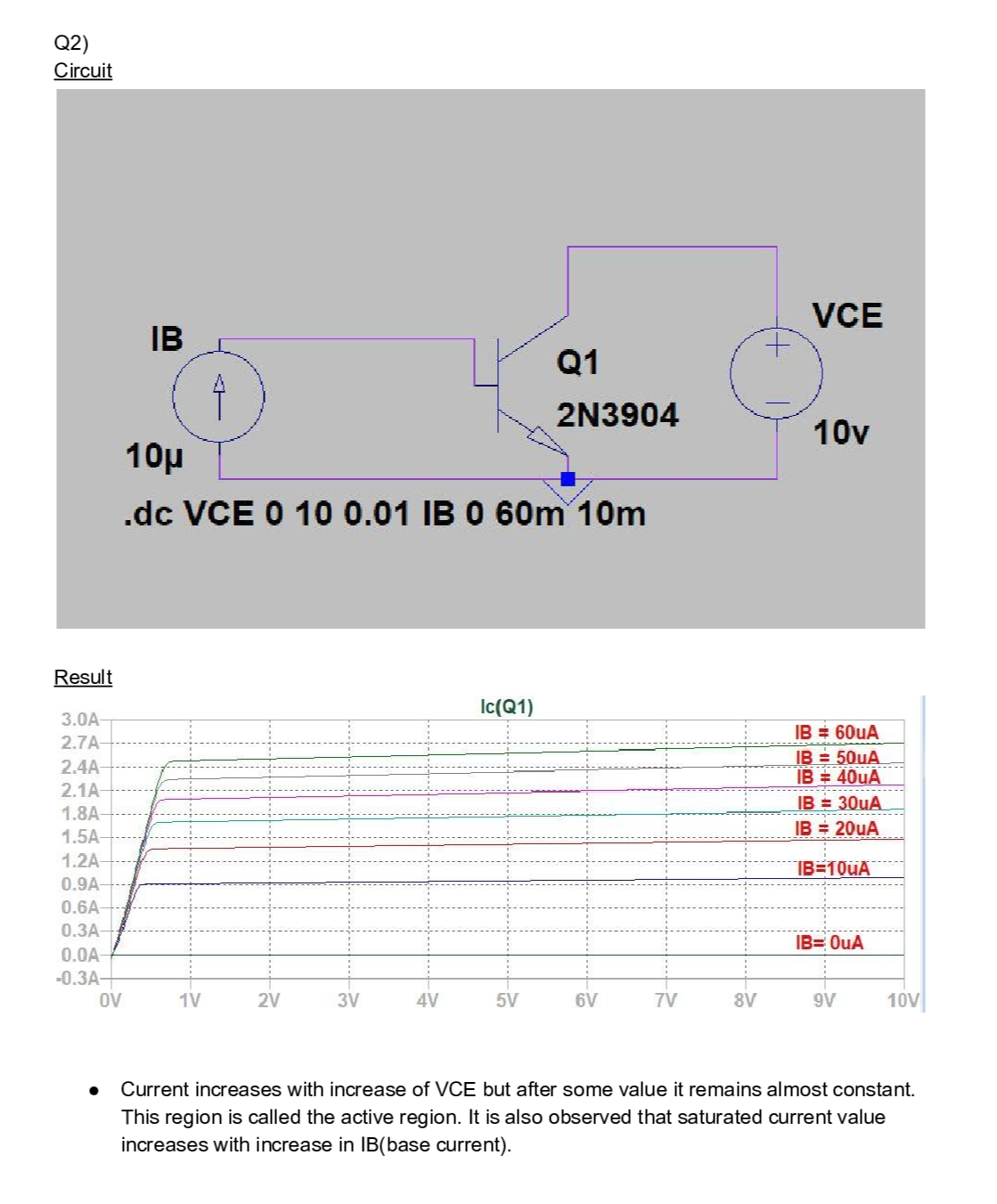Question

Embedded System

2. Using PSpice (or alternate), obtain the output characteristics (ic versus vce ) for an non 2N3904 BJT. The base-emitter junction is forward biased with a DC current source and the collector-base junction is reversed biased by a DC voltage source connected between the collector and emitter.

Use the DC analysis and set up the DC sweep. The dialog box should be opened and for the main Sweep variable type VCE , the linear sweep is set from 0 to 10 V in increments of 0.01 V. The Nested Seep button is clicked to open its dialog box, and for the Nested Sweep variable type iB , use a linear seep from 0 to 60m A in increment of 10 m A. In Probe add the trance IC(Q1) and obtain the BJT output characteristics(you are supposed to get the BJT's curves).Verified### Question 26890Embedded System

A Design a 1 mH toroidal inductor on a Carbonyl W core size T30.Determine the maximum wire size that could be used to realize a single layer winding.

### Question 26889Embedded System

In ADS, select a chip inductor from the Coil Craft library with an inductance value of 80nH. Determine the maximum Q factor of the

### Question 26888Embedded System

0. Using the inductor from Problem 8, determine the self resonant frequency of the inductor and comment on the maximum frequency in which the inductor may be used in a tuned circuit application.

### Question 26887Embedded System

Design an air core inductor with an inductance value of 84 nH. Use a copper wire of 0.050 inch diameter wound on a core diameter or0.100 inch. Determine the number of turns required assuming a tight spaced winding.

### Question 26886Embedded System

Model a chip resistor (size 0603) with a resistance of 50S in ADS.Consider an application in which 502 impedance must be maintained with +10%. Create a linear analysis and determine the maximum usable frequency of the chip resistor.

### Question 26885Embedded System

) Calculate the inductance of a 5 inch length of copper flat ribbon conductor. The dimensions of the ribbon are 0.100 inches in width and 0.002 inches thick.

### Question 26884Embedded System

Calculate the reactance of the wire from Problem 2 at 10 Hz, 10MHz, and 10 GHz. Create a Linear analysis in ADS and display the wire impedance vs. frequency

### Question 26883Embedded System

Calculate the wavelength of an electromagnetic wave operating at a frequency of 428MHZ.

### Question 26685Embedded System

. The MRF911D transistor with common-emitter S-Parameters for VCE=8V and IC=10 mA
! MRF911D.S2P
! MRF911
! VCE=5V; IC=20mA
# GHz S MA R 50
! S-PARAMETER DATA
\text { So for } 0.5 \mathrm{GHz}, \mathrm{S} 11=0.54 \angle-180, \mathrm{~S} 21=6.4 \angle 85, \mathrm{~S} 12=0.06 \angle 60 \text { , and } \mathrm{S} 22=0.19 \angle-70
Design 1GHZ narrowband amplifier. Use a matching circuit with L's and C's for input andoutput matching to 50 2. (33%)

### Question 26684Embedded System

Impedance match a complex load of ZL = 25+ j15 Q to a 50 Q source impedance using a three element L-C matching pi-type network with one series inductor. Remember to perform conjugate matching for maximum power transfer. Determine the component values for operation at 300 MHz. Simulate using ADS and include results of Sl1 and S21plots up to 1 GHz. Place a marker at 500 MHz. (33 pts)

### Submit query